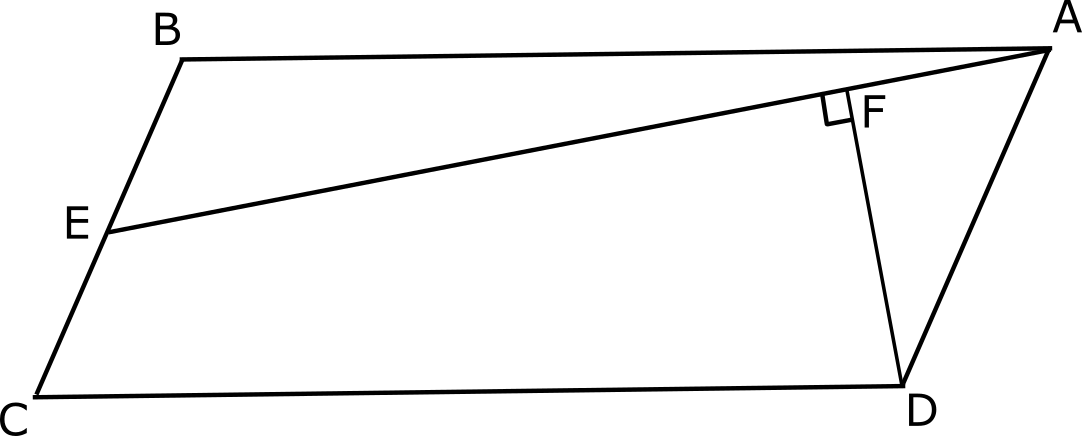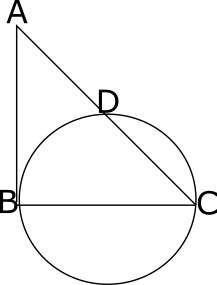# CAT Geometry Questions with Answers

#### Geometry

Geometry is a vast topic and more than 5 questions appear from Geometry every year in CAT. The important subtopics under geometry are triangles, similarities and congruencies, circles, properties of circles, polygons, and quadrilaterals. The following CAT geometry questions are provided with detailed explanations and video solutions to help students learn better.

### Take a free CAT Mock

Thousands of students have taken Cracku's Free CAT Mock.

Instructions

For the following questions answer them individually

Question 1

ABCD is a parallelogram, as shown in the figure, with AB = 4 cm and BC = 2 cm. E is the midpoint of BC. DF is the perpendicular drawn from D to AE. What is the length of CF?Question 2

In the given figure, BC is the diameter of the circle. AB is a tangent to the circle at point B. If the radius of the circle is 4 cm, then find the value of AC*DC?Question 3

Find the number of common tangents that can be drawn to the circles $$x^2+y^2=25$$ and $$(x-6)^2+(y-8)^2=25$$.

Question 4

Two lines: 14x - 33y + 15 = 0 and 28x - 66y + 52 = 0 are tangents to the same circle. If the center of the circle is in the second quadrant, find the radius of the circle.

Question 5

Find the number of points with integral coordinates which lie in the region enclosed by |x|+|y| = 4 or on the boundary.

Question 6

A rectangular field is 432 sqm in area. If the length is decreased by 4m and breadth is increased by 2m, a square ground can be formed. What would be the length of the side of the square ground?

Question 7

A pavement of width 0.5 metres was built around a square swimming pool and the area of the pavement is 49 sq metres. What is the area of the swimming pool?

Question 8

If the sides of a triangle are 7a+24b, 24a+7b and 25a+25b, where a and b are positive, which of the following is true?

Question 9

The co-ordinates of the triangle are A(3,4), B(-1,-2), C(1,4). Find the distance of the centroid from BC.

Question 10

There is a circle of radius 5 cm, in which is inscribed a quadrilateral. It is further known that 2 of the adjacent sides of the quadrilateral are 6 cm and 8 cm. Determine the maximum possible area of the quadrilateral.

Question 11

In a polygon, all the interior angles are either 90 degrees or 270 degrees. If the number of interior angles which are 90 degrees is 31, find the number of angles which are 270 degrees?

Question 12

Three people A, B and C are standing at the vertices of an equilateral triangle. They start travelling towards each other in such a way that A is always moving towards C, C is always moving towards B and B is always moving towards A at a speed of 15m/s. If the side of the triangle is 60m, find the difference between the length of the path travelled by A and the shortest distance between the starting and ending points.

Question 13

AB = 12 cm and BC = 16 cm are sides of a rectangle ABCD. A perpendicular is drawn from A to the diagonal BD which meets the BC at Y. What is the length of CY?

Question 14

Suppose the volume of a football is 700% more than the volume of a cricket ball. By how much percent is the surface area of the football greater than the cricket ball?

Question 15

An equilateral triangle and a square are inscribed in a circle. What is the ratio of the area of the triangle to the ratio of the square?

Question 16

A ladder is two feet taller than the wall it is resting against. If the lower end of the ladder is kept 10 feet away from the wall, the upper end of the ladder just touches the top of the wall. Find the height of the wall?

Question 17

What are the coordinates of the circumcenter of the triangle formed by joining the points (0,0),(16,6) and (10,-4)?

Question 18

In a triangle ABC, a line is drawn parallel to BC such that it intersects AB at D and AC at E. The ratio of AD:DB = 3:2. A line is now drawn such that it makes an angle of 30 degrees with AB and cuts BC produced at F. If DE produced cuts AF at H, find the ratio of area of triangle AHC to area of triangle FHC.

Question 19

4 chords AB,BC,CD and DA are drawn inside a circle such that the lengths of the chords are 14cm, 17cm, 18cm and 23cm respectively. What is the area of the quadrilateral so formed?

Question 20

On a semi-circle with diameter AB, chord CD is drawn parallel to the diameter. The ends of the diameter and chord are joined to form a trapezium. The length of the diameter is 8cm and the length of the non-parallel side is 2cm.Find the length of the chord.